Next: Accelerated Charges Up: Relativity and Electromagnetism Previous: Force on a Moving

# Electromagnetic Energy Tensor

Consider a continuous volume distribution of charged matter in the presence of an electromagnetic field. Let there be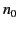particles per unit proper volume (that is, unit volume determined in the local rest frame), each carrying a charge. Consider an inertial frame in which the 3-velocity field of the particles is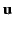. The number density of the particles in this frame is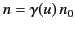. The charge density and the 3-current due to the particles are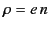and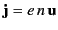, respectively. Multiplying Equation (1861) by the proper number density of particles,, we obtain an expression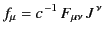(1863)

for the 4-force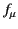acting on unit proper volume of the distribution due to the ambient electromagnetic fields. Here, we have made use of the definition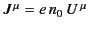. It is easily demonstrated, using some of the results obtained in the previous section, that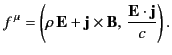(1864)

The previous expression remains valid when there are many charge species (e.g., electrons and ions) possessing different number density and 3-velocity fields. The 4-vector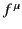is usually called the Lorentz force density.

We know that Maxwell's equations reduce to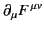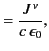(1865)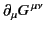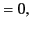(1866)

where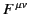is the electromagnetic field tensor, and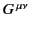is its dual. As is easily verified, Equation (1868) can also be written in the form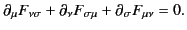(1867)

Equations (1865) and (1867) can be combined to give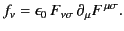(1868)

This expression can also be written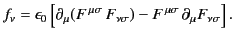(1869)

Now,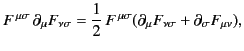(1870)

where use has been made of the antisymmetry of the electromagnetic field tensor. It follows from Equation (1869) that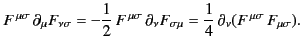(1871)

Thus,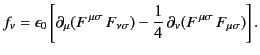(1872)

The previous expression can also be written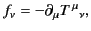(1873)

where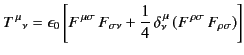(1874)

is called the electromagnetic energy tensor. Note that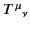is a proper-4-tensor. It follows from Equations (1778), (1781), and (1813) that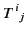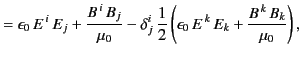(1875)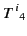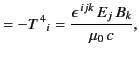(1876)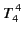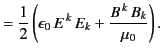(1877)

Equation (1875) can also be written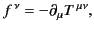(1878)

where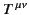is a symmetric tensor whose elements are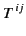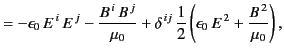(1879)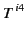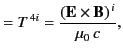(1880)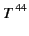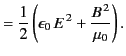(1881)

Consider the time-like component of Equation (1880). It follows from Equation (1866) that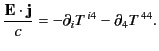(1882)

This equation can be rearranged to give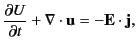(1883)

where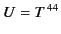and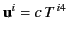, so that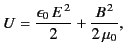(1884)

and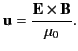(1885)

The right-hand side of Equation (1885) represents the rate per unit volume at which energy is transferred from the electromagnetic field to charged particles. It is clear, therefore, that Equation (1885) is an energy conservation equation for the electromagnetic field. (See Section 1.9.) The proper-3-scalar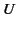can be identified as the energy density of the electromagnetic field, whereas the proper-3-vectoris the energy flux due to the electromagnetic field: that is, the Poynting flux.

Consider the space-like components of Equation (1880). It is easily demonstrated that these reduce to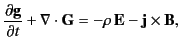(1886)

where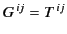and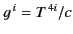, or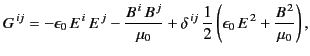(1887)

and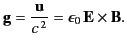(1888)

Equation (1888) is basically a momentum conservation equation for the electromagnetic field. (See Section 1.10.) The right-hand side represents the rate per unit volume at which momentum is transferred from the electromagnetic field to charged particles. The symmetric proper-3-tensorspecifies the flux of electromagnetic momentum parallel to the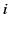th axis crossing a surface normal to the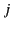th axis. The proper-3-vector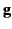represents the momentum density of the electromagnetic field. It is clear that the energy conservation law (1885) and the momentum conservation law (1888) can be combined together to give the relativistically invariant energy-momentum conservation law (1880).Next: Accelerated Charges Up: Relativity and Electromagnetism Previous: Force on a Moving
Richard Fitzpatrick 2014-06-27